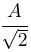# Active Reading Solution: The Standing Wave Equation

1. Plugging in t=T/4 turns this equation into y(x) = A sin(2px/l). At that instant the string looks like a sine wave with amplitude A and wavelength l. (At other times the wave has different amplitudes, but always the same wavelength.)

2. Plugging in x=l/8 turns this equation into y(t) =sin(2pt/T). That particular point on the string is oscillating with amplitude isand period T. (At other locations the wave has different amplitudes, but always the same period.)
And here comes a great video that Gary made!